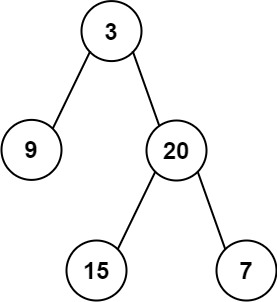# Leetcode 105 - Construct binary tree from preorder and inorder traversal

Note:

• The return value is the root, so we need to return node in the recursion function.
• The first element of preorder is the current root.
• Split inorder into left and right subtree by the value of root. (All vals are unique)
• Know how to assign root‘s left and right subtree, then by the length of left and right inorder, split preorder into left and right subtree.

Question:

Given two integer arrays preorder and inorder where preorder is the preorder traversal of a binary tree and inorder is the inorder traversal of the same tree, construct and return the binary tree.

Example:Code: